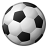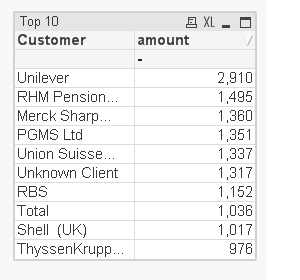# QlikView App Dev

Discussion Board for collaboration related to QlikView App Development.

Announcements
Skip the ticket, Chat with Qlik Support instead for instant assistance.
cancel
Showing results for
Did you mean:Creator

## Top 10 customers, linked to variables in a slider

Good day,

Hope all is well and hoping you can help me with a quick query

Basically I have the following calculation, that enables me to create a slider using variables, where you can choose the min and max customer amounts to include/exclude.

=Num(Sum({\$<Year_YYYY={'\$(=vMaxYear)'},Customer={"=Sum(amount)<=\$(vMinLimit) or Sum(amount)>=\$(vMaxLimit)"}>} amount)/1000,'#,###0')

I now want to create another table, just to show me a top 10, as per calculation below

=Num(sum({<Year_YYYY={'\$(vMaxYear)'},Customer = {'=rank(sum(amount),4)<=10'}>} amount)/1000,'#,###0')

I would like to put this top 10 ranking calc, into the above variables calculation, but am struggling to combine the two.

I attach a qvd for refrence

Any help, would be great.

1 Solution

Accepted SolutionsMVP

Try this expression

IF(

rank(total Sum({\$<Year_YYYY={'\$(=vMaxYear)'},Customer={"=Sum(amount)<=\$(vMinLimit) or Sum(amount)>=\$(vMaxLimit)"}>}amount)/1000)<=10,

Num(Sum({\$<Year_YYYY={'\$(=vMaxYear)'},Customer={"=Sum(amount)<=\$(vMinLimit) or Sum(amount)>=\$(vMaxLimit)"}>}amount)/1000,'#,###0')

)

6 RepliesMVP

Try this expression

IF(

rank(total Sum({\$<Year_YYYY={'\$(=vMaxYear)'},Customer={"=Sum(amount)<=\$(vMinLimit) or Sum(amount)>=\$(vMaxLimit)"}>}amount)/1000)<=10,

Num(Sum({\$<Year_YYYY={'\$(=vMaxYear)'},Customer={"=Sum(amount)<=\$(vMinLimit) or Sum(amount)>=\$(vMaxLimit)"}>}amount)/1000,'#,###0')

)Creator
Author

Hi Manish,

Many thanks for taking the time, to giving me some adviceI like the calc, but do you know why this removes the total amount, as you can see below?

ThanksMVP

Try

SUM(Aggr(IF(

rank(total Sum({\$<Year_YYYY={'\$(=vMaxYear)'},Customer={"=Sum(amount)<=\$(vMinLimit) or Sum(amount)>=\$(vMaxLimit)"}>}amount)/1000)<=10,

Num(Sum({\$<Year_YYYY={'\$(=vMaxYear)'},Customer={"=Sum(amount)<=\$(vMinLimit) or Sum(amount)>=\$(vMaxLimit)"}>}amount)/1000,'#,###0')

),Period_Sort,Period_Number,Month))MVP

Or if this is a straight table just use Sum of rows on the expressions tabCreator
Author

ahhh yes, something so simple yet leaves the brain sometimes haha

many thanks Sunny, very helpful as everCreator
Author

this was the pefect calc, appreciate the response, its very much appreciated.

Added into the sum expression from Sunny, it's all working

have a good weekend bothCommunity Browser